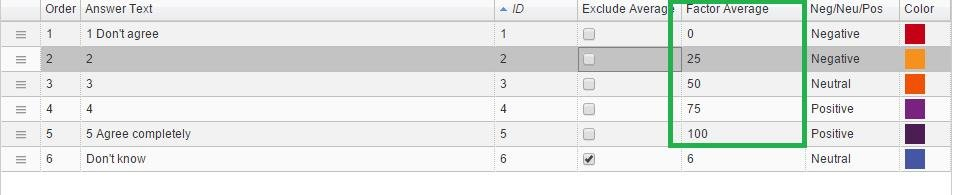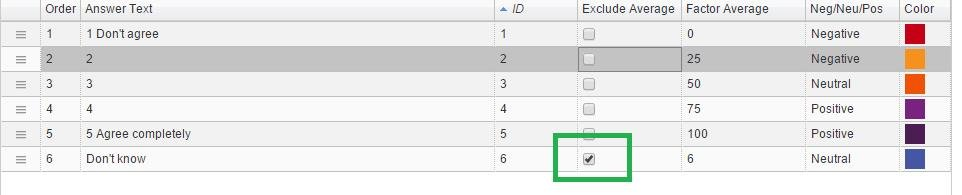In the correlation analysis, an administrator can define factors, exclude answers from the analysis and group answers:

## Factors

In Dapresy Pro, each answer has a defined factor. The image below shows an example of factors in an answer block with a scale of 1-5. The factor values have been set to 0, 25, 50, 75 and 100. These values are used instead of 1, 2, 3, 4 and 5 in the correlation calculations.## Valid respondents – “Don’t know”/”Missing values” in data

Only respondents that have valid answers in both questions are included in the calculation. If a respondent has a missing value or a non-valid answer in any of the two questions the respondent is not included in the calculation.

An answer is counted as a non-valid answer if it has been defined as an “Exclude from Average” in the Answer block page. An example of this is a “Don’t know” answer in a scale question, as shown below.## Grouped answers are not included in the calculations

If the selected questions contain Grouped answers, then these are ignored and not used in the calculation.

## Note: Test against an Indexes

The calculation of the correlation analyses is based on respondent level data. This means that Indexes created in Dapresy Pro cannot be used when performing a correlation test because Index data is not stored on a respondent level in the database.

If a correlation test should be made against an Index, then it can be made by using any of the following workarounds:

1) Calculate the index (per respondent) outside Dapresy Pro and include as a variable in the data import
2) Calculate the index (per respondent) in Dapresy Pro by using the compute variable function

Both options above will give an index result that is stored on a respondent level in the database. This means that a correlation test can be made against the index.